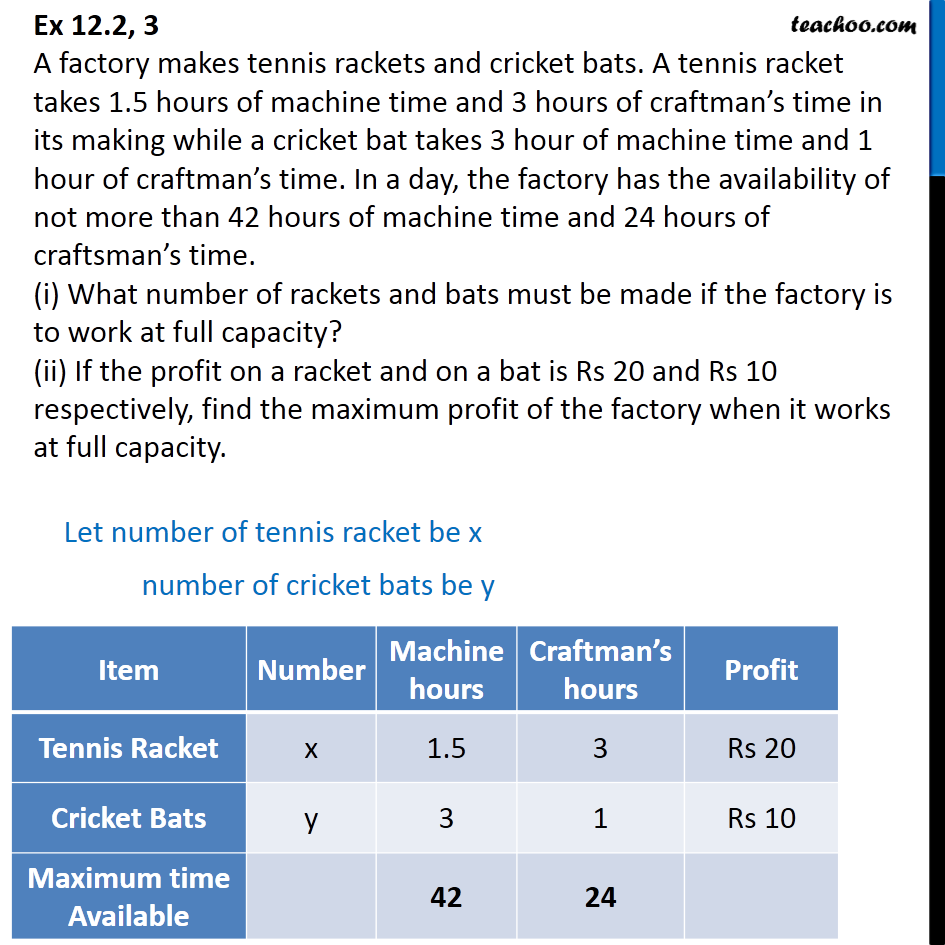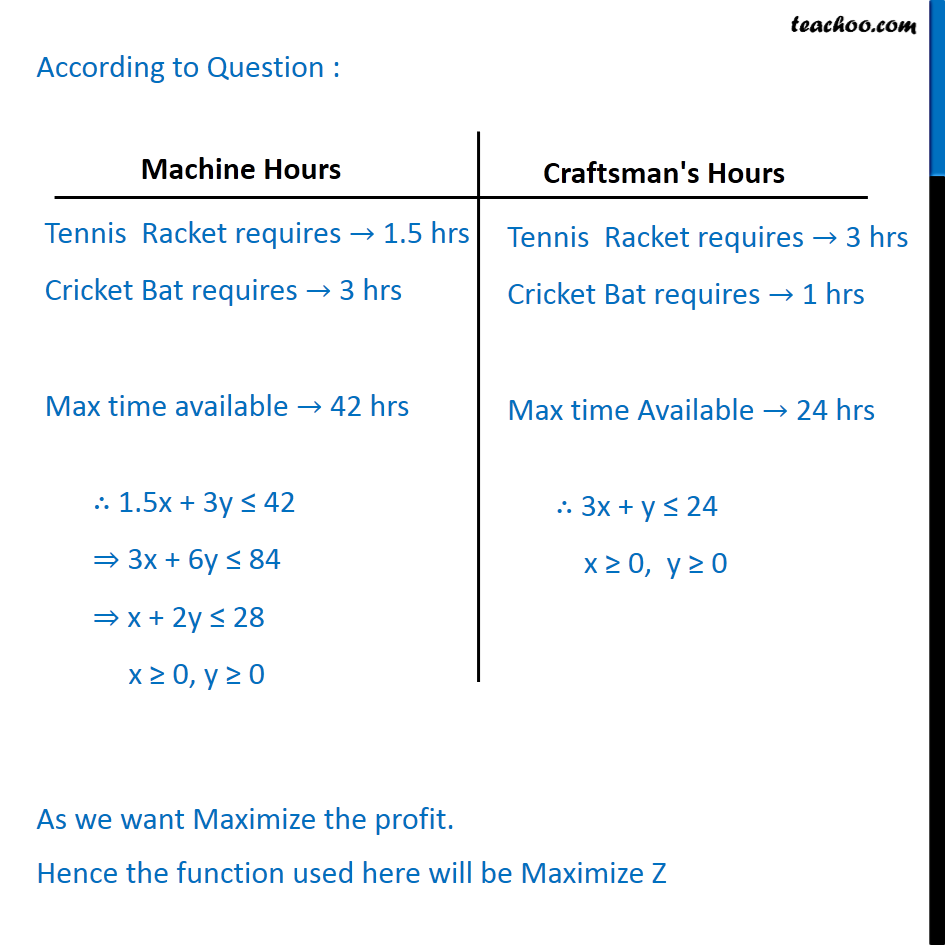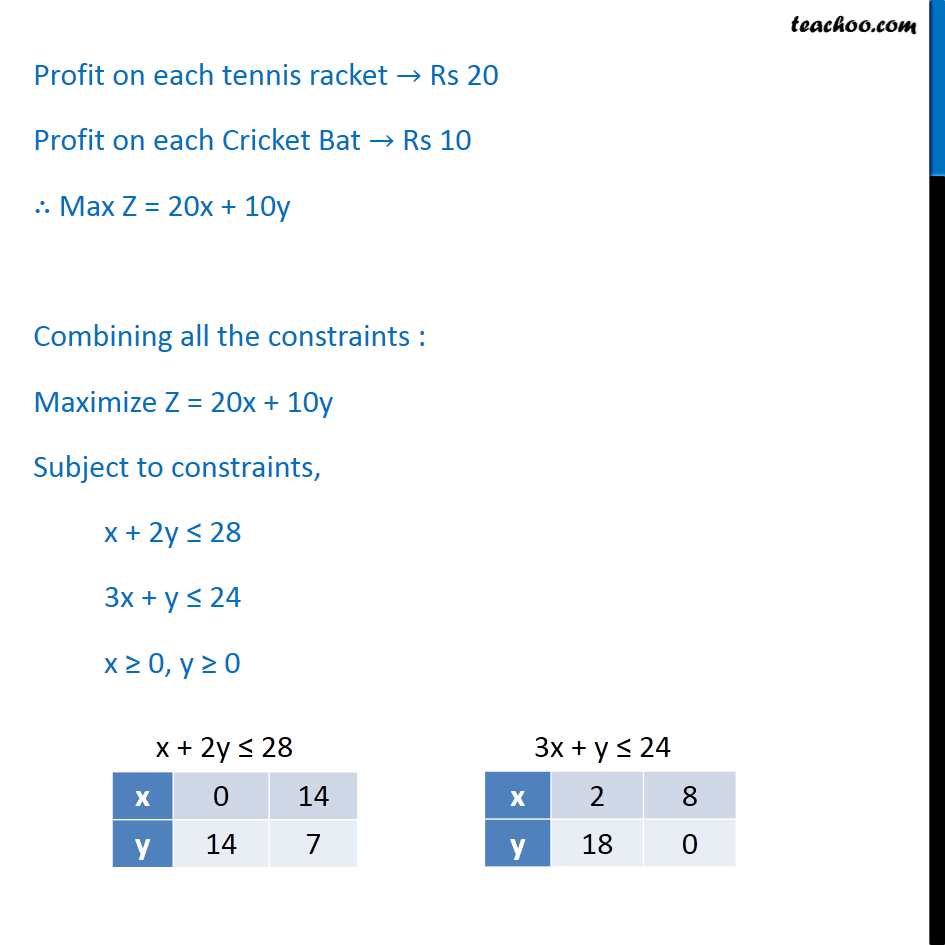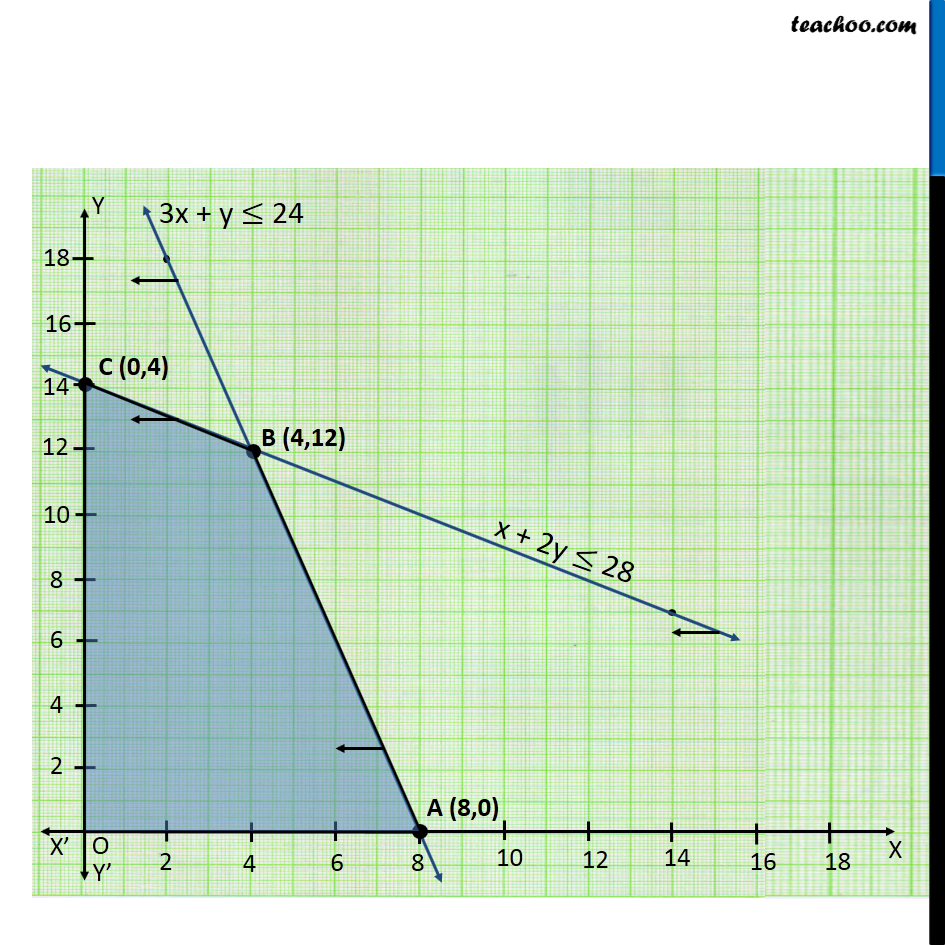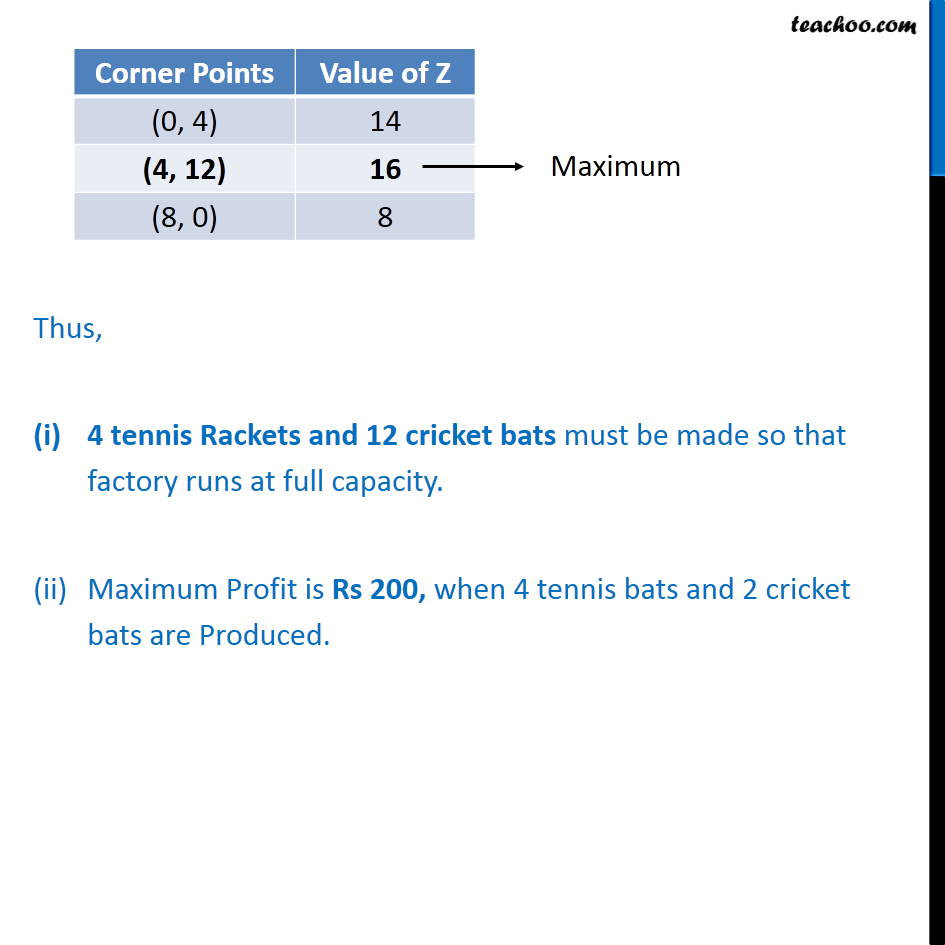1. Chapter 12 Class 12 Linear Programming
2. Serial order wise
3. Ex 12.2

Transcript

Ex 12.2, 3 A factory makes tennis rackets and cricket bats. A tennis racket takes 1.5 hours of machine time and 3 hours of craftman s time in its making while a cricket bat takes 3 hour of machine time and 1 hour of craftman s time. In a day, the factory has the availability of not more than 42 hours of machine time and 24 hours of craftsman s time. (i) What number of rackets and bats must be made if the factory is to work at full capacity? (ii) If the profit on a racket and on a bat is Rs 20 and Rs 10 respectively, find the maximum profit of the factory when it works at full capacity. Let number of tennis racket be x number of cricket bats be y According to Question : As we want Maximize the profit. Hence the function used here will be Maximize Z Profit on each tennis racket Rs 20 Profit on each Cricket Bat Rs 10 Max Z = 20x + 10y Combining all the constraints : Maximize Z = 20x + 10y Subject to constraints, x + 2y 28 3x + y 24 x 0, y 0 Thus, 4 tennis Rackets and 12 cricket bats must be made so that factory runs at full capacity. Maximum Profit is Rs 200, when 4 tennis bats and 2 cricket bats are Produced.

Ex 12.2